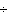Name:    5th Grade Math- Operations & Algebraic Thinking Std. 2 Practice Test A

Multiple Choice
Identify the choice that best completes the statement or answers the question.

Express the following calculations as an equations.

1.

Three times a number, increased by ten, is two.
 a. 10(3x)=2 c. (3x +10) -2 b. 2x + 10 =2 d. 3x+10=2

2.

10 times a number z decreased by 3 is 17.
 a. 10z-3=17 c. 10 x 3 -z =17 b. 10-3 +z =17 d. z x 10 +3 = 17

3.

The quotient of 10 and x is 6
 a. 10x=6 c. 1x + 10=6 b. 106=x d. 10x=6

4.

If a number x is added to 5 times y the result is 22.
 a. 5x + y = 22 c. 5y - x =22 b. 5y + x = 22 d. 5x - y =22

5.

If 10 is increased by the product of 4 and x, the result is 42.
 a. 10 - 4x = 42 c. 10 + 4x = 42 b. 4x - 10 =42 d. 10 + 4x =42

6.

Two times a number x decreased by 7 is 52.
 a. 2x - 7 = 52 c. 2x7 = 52 b. 2x + 7 = 52 d. 2x (7) = 52

7.

Ninety-nine is three times a number minus 1.
 a. 99 = 3+x - 1 c. 99 = 3x + 1 b. 99 = 1 - x(3) d. 99=3x-1

8.

The product of two numbers is 75.
 a. x + y = 75 c. x - y = 75 b. xy=75 d. xy = 75

9.

The quotient of two numbers is equal to the sum of those numbers.
 a. x + y = zy c. xy = xy b. xy = x - y d. xy = x + y

10.

The quotient of twenty and five more than a number is seventeen.
 a. 20    = 17x+5 c. 5 +20x= 17 b. 20x= 17 d. 20    = 175x